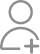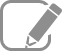Take Class 11 Tuition from the Best Tutors

•Affordable fees
•1-1 or Group class
•Flexible Timings
•Verified Tutors

Search in

# At what temperature is the root mean square speed of an atom in an argon gas cylinder equal to the rms speed of a helium gas atom at – 20 °C? (atomic mass of Ar = 39.9 u, of He = 4.0 u).

Learn Exercise 13Follow 1AnswerTemperature of the helium atom, THe = –20°C= 253 K Atomic mass of argon, MAr = 39.9 u Atomic mass of helium, MHe = 4.0 u Let, (vrms)Arbe the rms speed of argon. Let (vrms)Hebe the rms speed of helium. The rms speed of argon is given by: … (i) Where, R is the universal gas constant TAr...

Temperature of the helium atom, THe = –20°C= 253 K

Atomic mass of argon, MAr = 39.9 u

Atomic mass of helium, MHe = 4.0 u

Let, (vrms)Arbe the rms speed of argon.

Let (vrms)Hebe the rms speed of helium.

The rms speed of argon is given by:… (i)

Where,

R is the universal gas constant

TAr is temperature of argon gas

The rms speed of helium is given by:… (ii)

It is given that:

(vrms)Ar = (vrms)He= 2523.675 = 2.52 × 103 K

Therefore, the temperature of the argon atom is 2.52 × 103 K.

Now ask question in any of the 1000+ Categories, and get Answers from Tutors and Trainers on UrbanPro.com

Find Grade 11 Tuition near you

Looking for Class 11 Tuition ?

Learn from the Best Tutors on UrbanPro

Are you a Tutor or Training Institute?

Join UrbanPro Today to find students near you
X

### Looking for Class 11 Tuition Classes?

The best tutors for Class 11 Tuition Classes are on UrbanPro

• Select the best Tutor
• Book & Attend a Free Demo
• Pay and start Learning### Take Class 11 Tuition with the Best Tutors

The best Tutors for Class 11 Tuition Classes are on UrbanPro